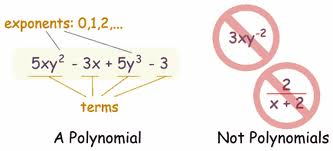# Adding Polynomials: Examples & Concept

Instructor: Jennifer Beddoe

Jennifer has an MS in Chemistry and a BS in Biological Sciences.

Addition of polynomials is as simple as combining like terms; however, there are still some pitfalls you might encounter along the way. This lesson shows you two ways to add polynomials and features a quiz so you can practice what you've learned.

## What is a Polynomial?

A polynomial is a mathematical expression consisting of constants, variables and exponents. It comes from the Latin 'poly' meaning 'many' and 'nomial' meaning 'terms,' so a polynomial has many terms; however, it can have many terms or only 1 term. A term can be a constant (number) or a variable (letter) and may or may not contain exponents. A polynomial cannot contain a fraction with a variable in the denominator or a negative exponent.Polynomial expressions are used throughout math and science. They provide scientists a way to solve problems - everything from the simplest mathematical word problem to complex scientific expressions. Probably the most famous polynomial expression is:Polynomials can be added, subtracted, multiplied or divided, just like mathematical equations with numbers. The process is the same, with just a few additional steps.

The addition of two or more polynomials is just a matter of combining like terms. Like terms are those that have the same variables and exponents. The coefficients in front of the variables may be different. It's the same as saying 'John has 3 apples.' The coefficient (3) describes how many apples John has, just as saying 5x means that there are five xs.

To solve the simplified problem below, you also need to combine like terms.

• I have 2 cats and 3 dogs, and Janet has 1 dog and 6 cats. Altogether, how many dogs and cats do we have?

You wouldn't add all the animals together, because dogs and cats are different. Rather, combined, Janet and I have 8 cats and 4 dogs.

The biggest area of confusion when adding polynomials is the pesky negative sign. It is important to add carefully so as to not miss the negative; 3 + 7 results in an entirely different answer than 3 + (-7).

There are two methods for adding polynomials. Either one will get you to the correct answer. The method you choose is really just a matter of which one makes more sense to you.

The first method for adding polynomials is the vertical method. In this method, you simply line up the like terms and then add. Don't forget to leave a space for the terms that do not match up with any other term. Otherwise, they can be easily overlooked or forgotten.

To unlock this lesson you must be a Study.com Member.

### Register to view this lesson

Are you a student or a teacher?